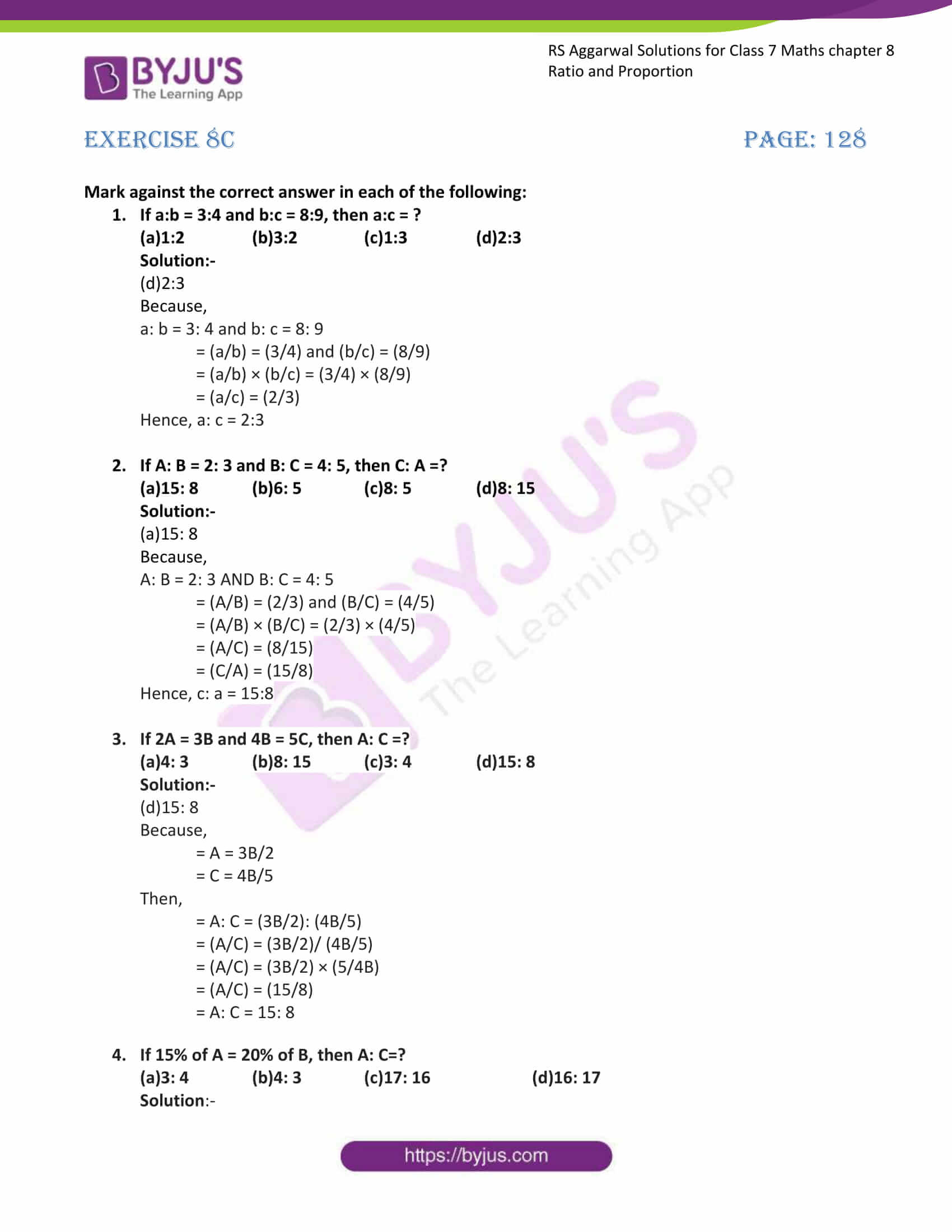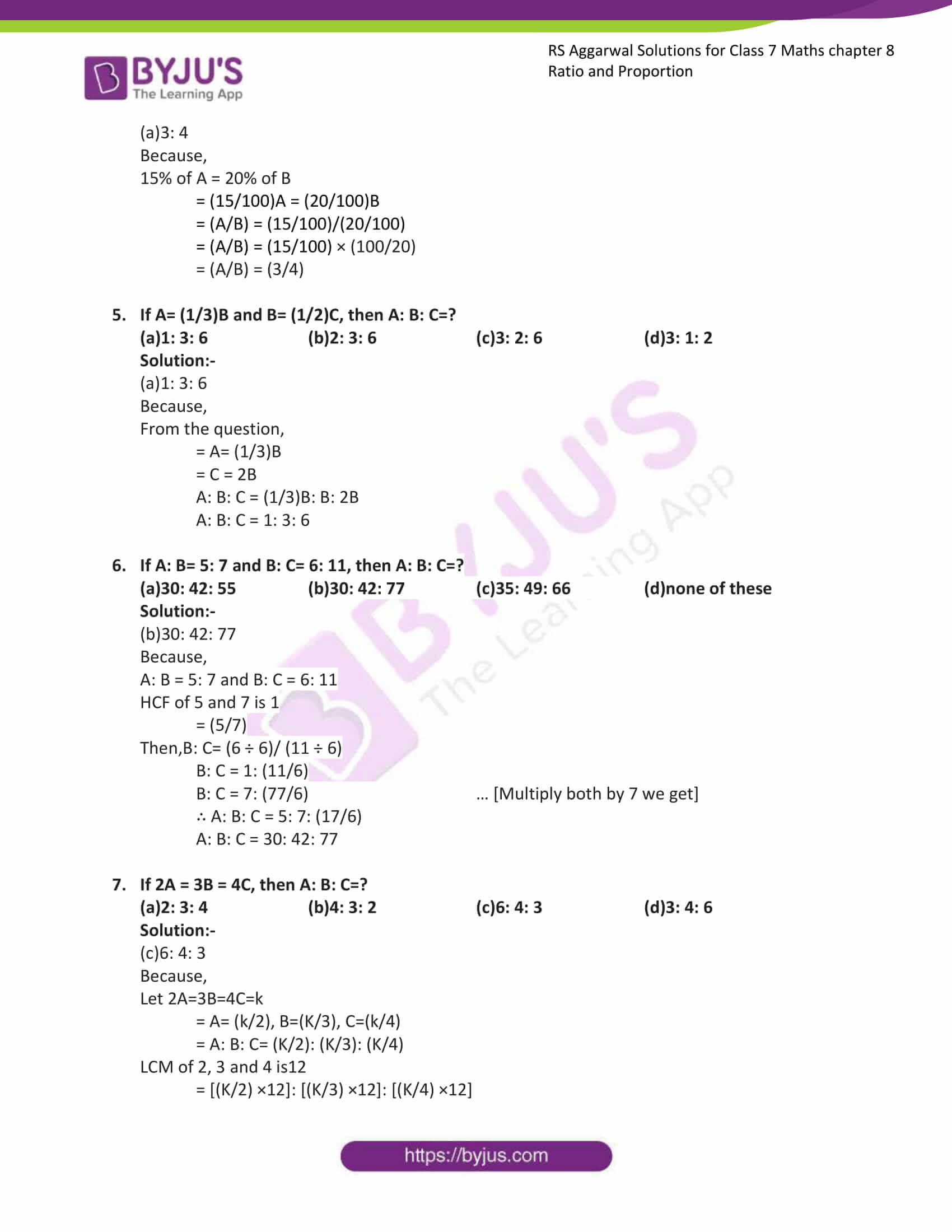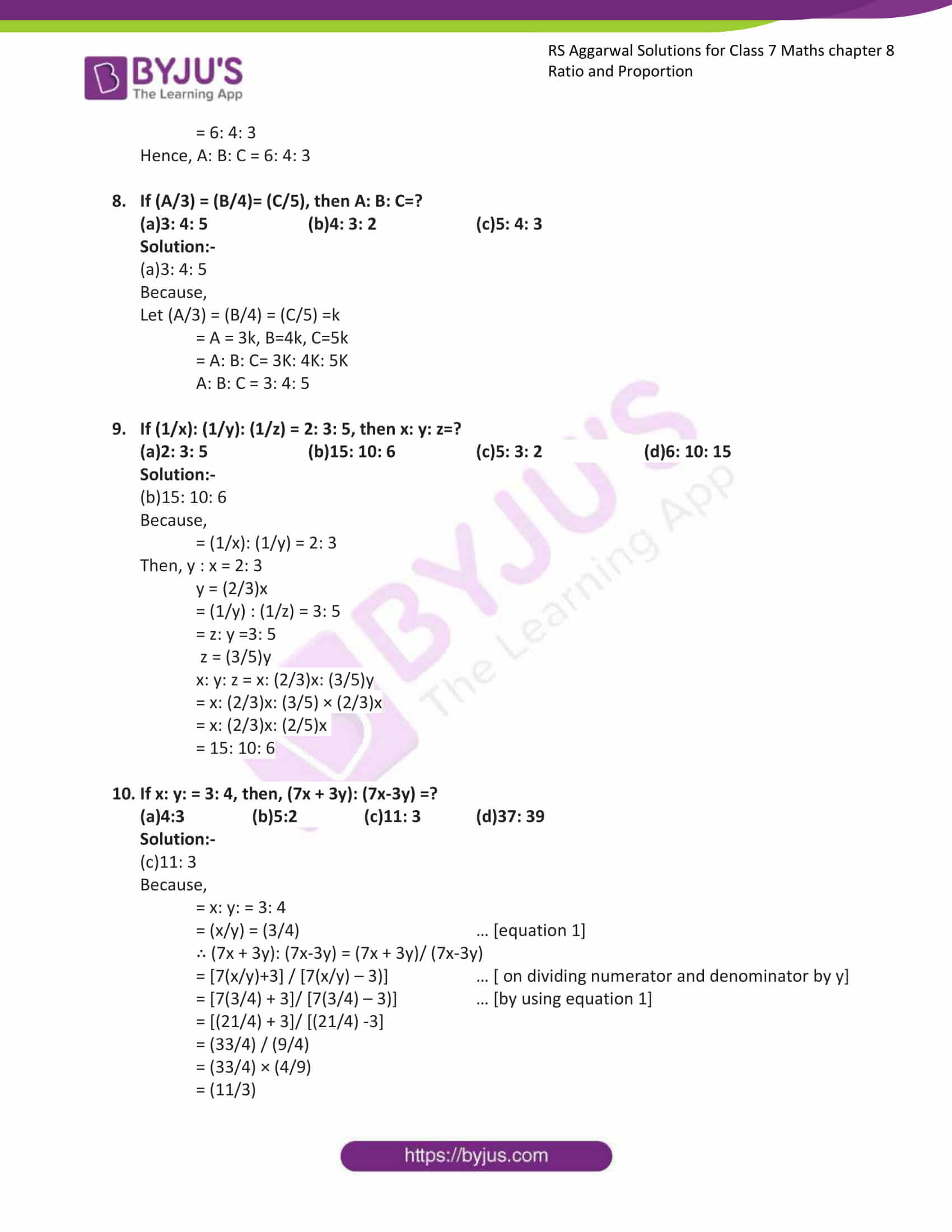# RS Aggarwal Solutions for Class 7 Maths Exercise 8C Chapter 8 Ratio and Proportion

RS Aggarwal Solutions for Class 7 Maths Exercise 8C Chapter 8 Ratio and Proportion, this exercise contains objective types of questions. The questions formed are related to the comparison of ratios and express the given ratios in a simple form. RS Aggarwal Solutions for Class 7 Chapter 8 is the best material for students who want to score good marks in Maths. Our specialist teachers have prepared RS Aggarwal Solutions for Class 7 Maths Chapter 8 Ratio and Proportion.

## Download the PDF of RS Aggarwal Solutions For Class 7 Maths Chapter 8 Ratio and Proportion – Exercise 8C### Access answers to Maths RS Aggarwal Solutions for Class 7 Chapter 8 – Ratio and Proportion Exercise 8C

Mark against the correct answer in each of the following:

1. If a:b = 3:4 and b:c = 8:9, then a:c = ?

(a)1:2 (b)3:2 (c)1:3 (d)2:3

Solution:-

(d)2:3

Because,

a: b = 3: 4 and b: c = 8: 9

= (a/b) = (3/4) and (b/c) = (8/9)

= (a/b) × (b/c) = (3/4) × (8/9)

= (a/c) = (2/3)

Hence, a: c = 2:3

2. If A: B = 2: 3 and B: C = 4: 5, then C: A =?

(a)15: 8 (b)6: 5 (c)8: 5 (d)8: 15

Solution:-

(a)15: 8

Because,

A: B = 2: 3 AND B: C = 4: 5

= (A/B) = (2/3) and (B/C) = (4/5)

= (A/B) × (B/C) = (2/3) × (4/5)

= (A/C) = (8/15)

= (C/A) = (15/8)

Hence, c: a = 15:8

3. If 2A = 3B and 4B = 5C, then A: C =?

(a)4: 3 (b)8: 15 (c)3: 4 (d)15: 8

Solution:-

(d)15: 8

Because,

= A = 3B/2

= C = 4B/5

Then,

= A: C = (3B/2): (4B/5)

= (A/C) = (3B/2)/ (4B/5)

= (A/C) = (3B/2) × (5/4B)

= (A/C) = (15/8)

= A: C = 15: 8

4. If 15% of A = 20% of B, then A: C=?

(a)3: 4 (b)4: 3 (c)17: 16 (d)16: 17

Solution:-

(a)3: 4

Because,

15% of A = 20% of B

= (15/100)A = (20/100)B

= (A/B) = (15/100)/(20/100)

= (A/B) = (15/100) × (100/20)

= (A/B) = (3/4)

5. If A= (1/3)B and B= (1/2)C, then A: B: C=?

(a)1: 3: 6 (b)2: 3: 6 (c)3: 2: 6 (d)3: 1: 2

Solution:-

(a)1: 3: 6

Because,

From the question,

= A= (1/3)B

= C = 2B

A: B: C = (1/3)B: B: 2B

A: B: C = 1: 3: 6

6. If A: B= 5: 7 and B: C= 6: 11, then A: B: C=?

(a)30: 42: 55 (b)30: 42: 77 (c)35: 49: 66 (d)none of these

Solution:-

(b)30: 42: 77

Because,

A: B = 5: 7 and B: C = 6: 11

HCF of 5 and 7 is 1

= (5/7)

Then,B: C= (6 ÷ 6)/ (11 ÷ 6)

B: C = 1: (11/6)

B: C = 7: (77/6) … [Multiply both by 7 we get]

∴ A: B: C = 5: 7: (17/6)

A: B: C = 30: 42: 77

7. If 2A = 3B = 4C, then A: B: C=?

(a)2: 3: 4 (b)4: 3: 2 (c)6: 4: 3 (d)3: 4: 6

Solution:-

(c)6: 4: 3

Because,

Let 2A=3B=4C=k

= A= (k/2), B=(K/3), C=(k/4)

= A: B: C= (K/2): (K/3): (K/4)

LCM of 2, 3 and 4 is12

= [(K/2) ×12]: [(K/3) ×12]: [(K/4) ×12]

= 6: 4: 3

Hence, A: B: C = 6: 4: 3

8. If (A/3) = (B/4)= (C/5), then A: B: C=?

(a)3: 4: 5 (b)4: 3: 2 (c)5: 4: 3

Solution:-

(a)3: 4: 5

Because,

Let (A/3) = (B/4) = (C/5) =k

= A = 3k, B=4k, C=5k

= A: B: C= 3K: 4K: 5K

A: B: C = 3: 4: 5

9. If (1/x): (1/y): (1/z) = 2: 3: 5, then x: y: z=?

(a)2: 3: 5 (b)15: 10: 6 (c)5: 3: 2 (d)6: 10: 15

Solution:-

(b)15: 10: 6

Because,

= (1/x): (1/y) = 2: 3

Then, y : x = 2: 3

y = (2/3)x

= (1/y) : (1/z) = 3: 5

= z: y =3: 5

z = (3/5)y

x: y: z = x: (2/3)x: (3/5)y

= x: (2/3)x: (3/5) × (2/3)x

= x: (2/3)x: (2/5)x

= 15: 10: 6

10. If x: y: = 3: 4, then, (7x + 3y): (7x-3y) =?

(a)4:3 (b)5:2 (c)11: 3 (d)37: 39

Solution:-

(c)11: 3

Because,

= x: y: = 3: 4

= (x/y) = (3/4) … [equation 1]

∴ (7x + 3y): (7x-3y) = (7x + 3y)/ (7x-3y)

= [7(x/y)+3] / [7(x/y) – 3)] … [ on dividing numerator and denominator by y]

= [7(3/4) + 3]/ [7(3/4) – 3)] … [by using equation 1]

= [(21/4) + 3]/ [(21/4) -3]

= (33/4) / (9/4)

= (33/4) × (4/9)

= (11/3)

### Access other exercises of RS Aggarwal Solutions For Class 7 Chapter 8 – Ratio and Proportion

Exercise 8B Solutions